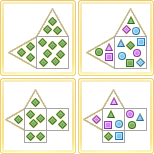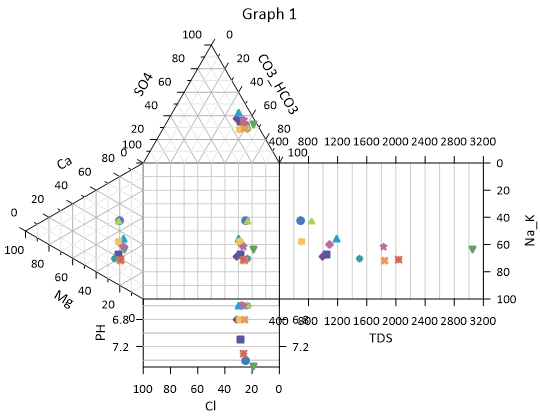# Durov and Durov Class Plots

 Click the Home | New Graph | Ternary | Durov Plot command to create a Durov plot. Click the Home | New Graph | Ternary | Durov Class Scatter Plot command to create a Durov plot with classed symbols. Click the Home | New Graph | Ternary | Extended Durov Plot to create a Durov plot with two extended scatter plots. Click the Home | New Graph | Ternary | Extended Durov Class Scatter Plot to create a Durov plot with two extended scatter plots and classed symbols. A Durov plot is one way of visualizing the chemistry of a rock, soil, or water sample. The Durov plot is very similar to the piper diagram. A Durov plot shows the relative concentrations of six ion groups in two ternary plots. A ternary diagram on the left represents the cations: calcium, sodium + potassium, and magnesium. A ternary diagram on the top represents the anions: chloride, carbonate + bicarbonate, and sulfate. A square plot is a projection of the cation and anion ternary plots. In an extended Durov plot, a pH plot is added to the bottom and a TDS (total dissolved solids) plot is added to the right side of the diagram. Click the Durov Plot, Durov Class Scatter Plot, Extended Durov Plot, Extended Durov Class Scatter Plot button to create a Durov plot.To plot data on a Durov plot, the sum of the three cationic data points (X + Y + Z) and the sum of the three anionic data points must each equal 100 percent. If the sum does not equal 100 percent Grapher normalizes the data when it is plotted. Once this relationship has been established between the data points, you need only know the values of any two data points in order to determine the third. Reading a point on a ternary diagrams can be done by looking at the location of each point as it relates to the three axes.

You can add a line connecting the points within each ternary diagram and the three plots. The line connects the data in the order it appears in the data file. When a line is added to one of the component plots in the Durov plot, a line is added to the other plots as well. To add a line, select any of the plots, open the Line tab in the Property Manager, and then select a line style other than Invisible. A line is displayed in all three or five plots.

When creating a Durov plot, Grapher assigns the data file columns in the following order: cation X, cation Z, cation Y, anion X, anion Z, anion Y, pH (if applicable), TDS (if applicable),and class (if applicable). If your data is ordered differently, select the appropriate columns by selecting the Anion or Cation ternary plot or pH or TDS scatter plot and specifying columns in the Property Manager Plot page.Durov plots show ionic concentrations in water, soil, or rock chemical samples.

## Creating a New Durov Plot

The following steps demonstrate how to create a piper diagram:

1. Click the Durov Plot, Durov Class Scatter Plot, Extended Durov Plot, or Extended Durov Class Scatter Plot command.
2. Select the data file in the Open Worksheet dialog. Select a new data file, or select a file that is already open in the Open worksheets section of the dialog.
3. Click Open to create the Durov plot.

## Editing Plot Properties

To change the features of a Durov plot, including the columns used to create the diagram, open properties by selecting the anion ternary plot, cation ternary plot, square plot, pH plot, or TDS plot and editing the properties in the Property Manager.

Label properties are edited independently for the plots in the Durov diagram. However, label properties can be applied to the Durov plot components at the same time by selecting the anion, cation, square, pH, and/or TDS plots and editing the similar properties in the Property Manager.

The Worksheet, Class column, data limits, symbol, and line properties are shared between the plots. The property is changed for all plots when the property is changed for any one plot. The anion and cation plots have Data Limits Properties properties for clipping and setting a data filter.

The extended or standard format is specified on the Graph page.

Click the following tabs in the Property Manager to change different properties: